output.to from Sideway
Draft for Information Only

# Content

```  Acoustic Plane Wave   Complex Exponential Representation   Plane Wave Properties   Medium Acoustic Impedance   Plane Wave Superimposition```

## Acoustic Plane Wave

For a 1D acoustic plane wave in x direction,:where

p is a function of x and t.
c is the speed of propagation.

The general solution of the equation is an arbitrary wave propagation along positive x direction and negative x direction.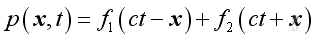For ct-x, the propagation of pressure fluctuation should be repeated after the period ct-x, therefore after time t, the wave should propagate a distance x in positive direction outwardly.

But for ct+x, the propagation of pressure fluctuation should be repeated after the period ct+x, therefore after time t, the wave should propagate a distance x in negative direction inwardly.

Since p is a function of x and t, it is characteristic by a double periodicity.

In time domain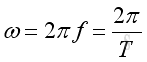where

ω is angular velocity
ƒ is frequency
ωT is period

And in physical domain: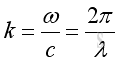where

k is wave number
c is speed of wave propagation λ is wave length

Consider a solution of the form: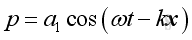where

ω is the angular frequency
k=ω/c
a1 is the magnitude constant, therefore: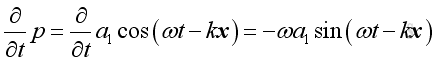,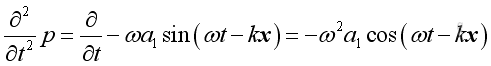and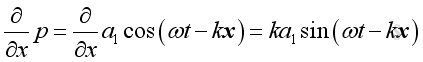,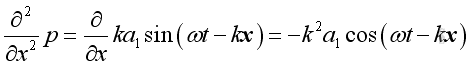Substituting into the 1D wave equation and the form of solution is confirmed.
And a solution of the same form in negative x direction is: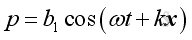Therefore the general harmonic wave solution of wave equation is: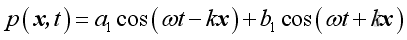Similarly, consider a solution of the form: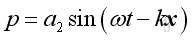where

ω is the angular frequency
k=ω/c,
a2 is the magnitude constant,
therefore: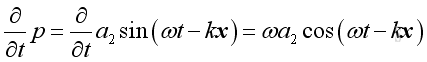,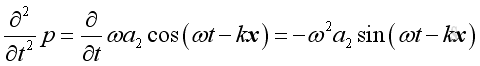and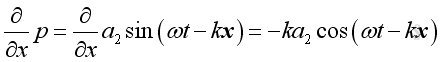,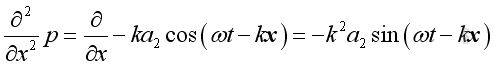Substituting into the 1D wave equation and the form of solution is confirmed also.

Since the 1D wave equation is linearized by making the differential coefficient is of first order only, the sum of two solution forms is also a solution of the wave equation. The differences between two solutions are the phase angle and the magnitude.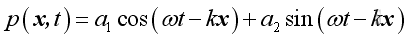The general expression of a harmonic function is: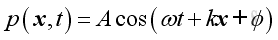and it can be decomposed into sinusoidal and cosinusoidal components in quadrature of 90 degrees out of phase: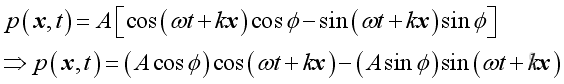These two components can be individual harmonic function with arbitrary phase angle φ as in the first two solutions. Or they can be linked solution in a linear system in which the phase angle is not an arbitrary and is related as in the above solution:

## Complex Exponential Representation

Since in complex system,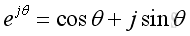The solution of wave equation can be expressed in a complex exponential form,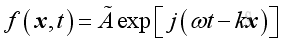where

Ã is Complex function of the form a+jb
exp[j(ωt-kx)] is ej(ωt-kx)
j is √(-1)

Since it is a complex function, the real acoustic pressure function can be represented by the real part of the complex expression.
Therefore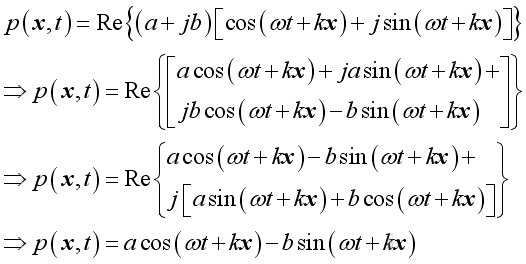By comparing with the wave equation solution, imply: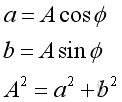Therefore, the general complex harmonic wave solution of 1D wave equation is :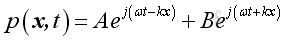## Plane Wave Properties

Consider a plane wave propagates along positive x direction: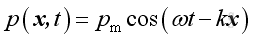or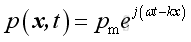From the linearized conservation of momentum, the relationship between acoustic pressure and acoustic velocity is: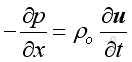Therefore: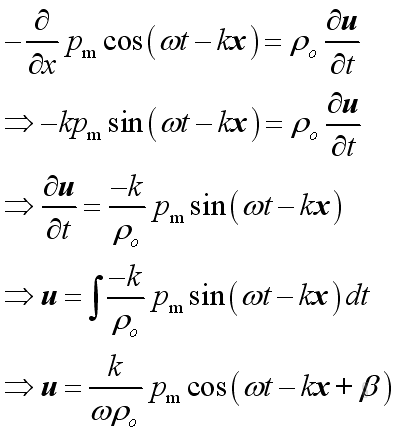The differences in magnitude between acoustic pressure and acoustic velocity are the relative magnitude and the relative phase angle.
Wave propagation is a kind of forced vibration, from the linearized conservation of momentum,  Then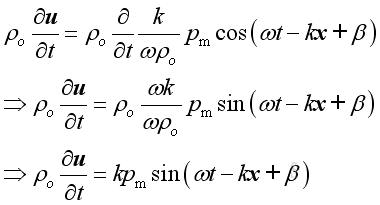or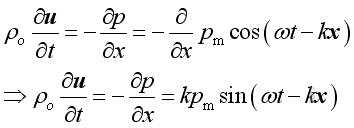Imply :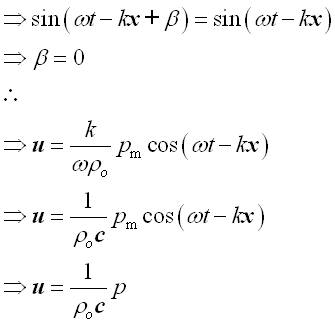Therefore the relationship between acoustic pressure and acoustic velocity is: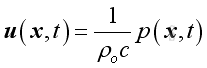In general: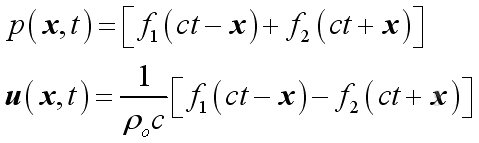## Medium Acoustic Impedance

The acoustic impedance of a medium is defined as the ratio of acoustic pressure to the acoustic velocity, Then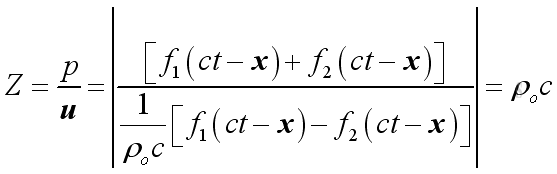For air, z=400

## Plane Wave Superimposition

Consider two harmonic plane waves propagate of the same frequency along positive x direction and negative x direction respectively. The pressure fluctuation of two harmonic plane waves i.e. A along positive x direction and B along negative x direction are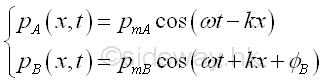If the two harmonic plane waves are in phase at x=0, then the phase angle at x is equal to zero also. Therefore the pressure fluctuation of two harmonic plane waves at x are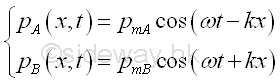The total sound pressure at x is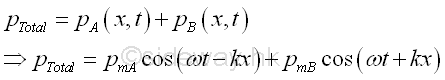According to the linearized equation of motion,Imply,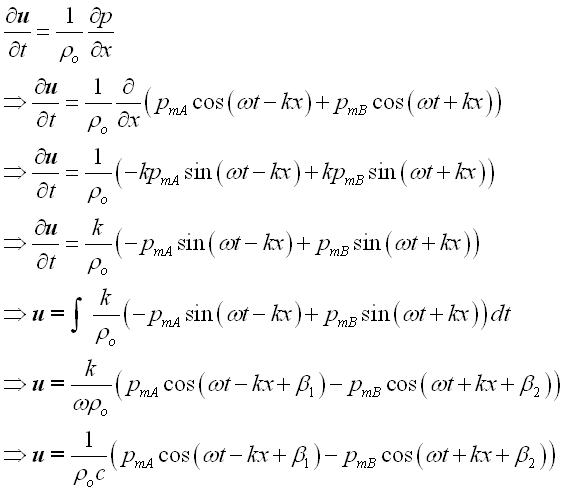From the linearized equation of motion, imply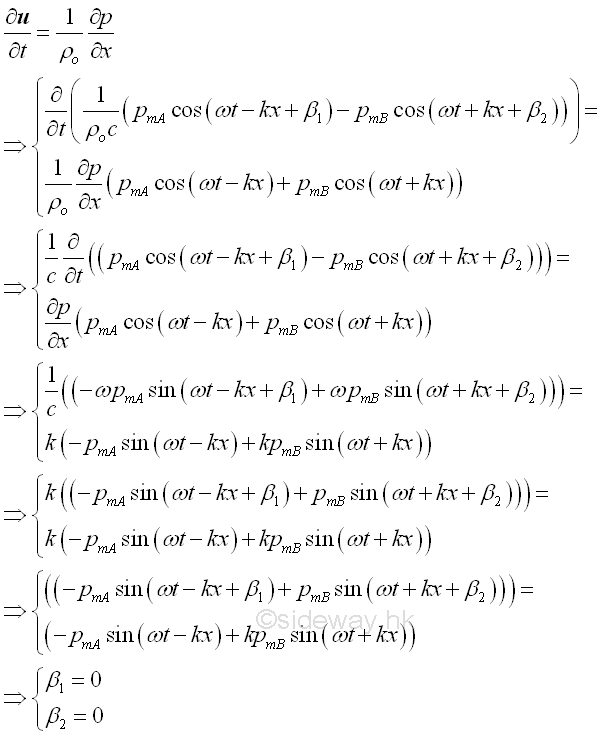Therefore, the vector sum of the particle velocity at x is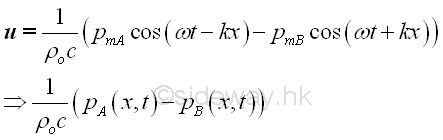ID: 100900021 Last Updated: 30/7/2012 Revision: 2 Ref:References

1. Michael P. Norton; Denis G. Karczub,, 2003
2. G. Porges, 1977
3. Douglas D. Reynolds, 1981
5. F. Fahy, 2001
6. D.A. Biew; C.H. Hansen, 1996Home 5

Management

HBR 3

Information

Recreation

Culture

Chinese 1097

English 337

Computer

Hardware 149

Software

Application 187

Numeric 19

Programming

Web 757

CSS 1

HTML

Knowledge Base

OS 389

MS Windows

Knowledge

Mathematics

Algebra 20

Geometry 18

Calculus 67

Engineering

Mechanical

Rigid Bodies

Statics 92

Dynamics 37

Control

Acoustics 19

Physics

Electric 10